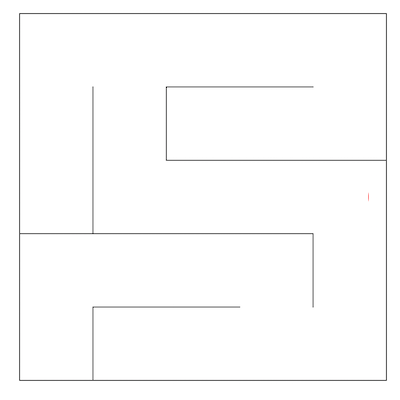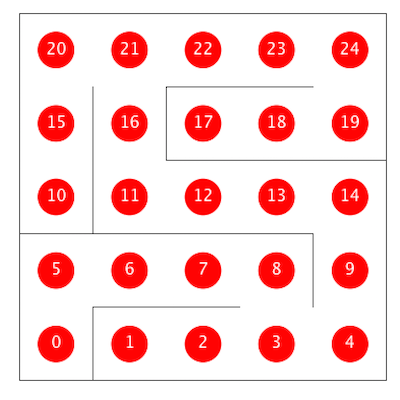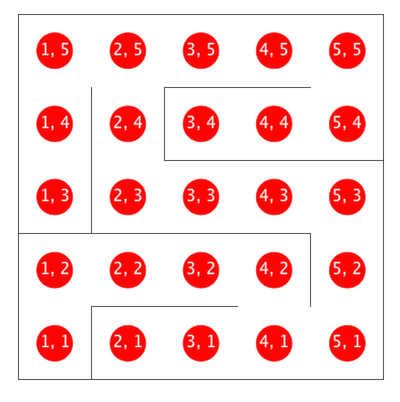Lab 13: Graphs

Attendance is not required for this week. Autograder will not be available until Friday, 4/24.

A. Introduction to our Maze Solver

In this lab, we'll explore how various graph algorithms behave in the context of mazes, like the one shown below.One way to represent a maze is as an undirected graph. The vertices of such a graph are shown below, with one dimensional (vertex number) coordinates on the left version and X/Y coordinates on the right version. If there is no wall between two adjacent vertices, then the corresponding graph has an undirected edge between the vertices. For example, adj(11) would be an iterable containing the integers 12 and 16.In this lab, you'll implement a few key graph algorithms using the provided Maze class, which has the following methods of interest:

public int N(): Size of the maze (mazes are N x N)
public int V(): Total number of vertices in the maze
public Iterable<Integer> adj(int v): Returns the neighbors of v
public int toX(int v): Returns the x coordinate of vertex v
public int toY(int v): Returns the y coordinate of vertex v
public int xyTo1D(int x, int y): Returns the vertex number of x, y

We've provided MazeDepthFirstPaths, a version of DepthFirstPaths adapted for use with mazes. For official lab credit, you'll need to implement at least an adaptation of BreadthFirstPaths, and for those of you want a deeper understanding of graph algorithms, we've also provided challenges to write a cycle detection algorithm as well as to implement the A* shortest paths algorithm. Specifically, you'll end up with:

• MazeDepthFirstPaths.java: Uses DFS to find all paths from a given source, terminating as soon as the target vertex is observed. Provided for you.
• MazeBreadthFirstPaths.java: Uses BFS to find all paths from a given source, terminating as soon as the target vertex is observed.
• MazeCycles.java: Searches for cycles in the maze. If a cycle is detected, the algorithm terminates.
• AStarPath.java: Searches for the shortest path from source to a target using A*, terminating when the target is observed.

These four maze solvers should be subclasses of the abstract MazeExplorer class, which has the following fields and methods:

public boolean[] marked: Locations to mark in blue
public int[] distTo: Distances to draw over the Maze
public int[] edgeTo: Edges to draw on Maze
public Maze maze: The maze being solved
public void announce(): Call whenever you want the drawing updated
public solve(): Solves the given Maze problem

The Maze class has special functionality built in so that it can see your MazeExplorer's public variables. Specifically, whenever you call announce, it will draw the contents of your MazeExplorer's marked, distTo, and edgeTo arrays. Make sure to call the announce method any time you want the drawing to be updated.

As an example, try compiling and running TrivialMazeExplorerDemo.java. Open up the TrivialMazeExplorer and TrivialMazeExplorerDemo files to understand what's going on.

As a more complex example, try compiling and running DepthFirstDemo. This code was adapted from the DepthFirstPaths class that was discussed during lecture.

If you want to tweak the parameters of the maze, you can edit the maze.config file. There are three different types of mazes (SINGLE_GAP, POPEN_SOLVABLE, and BLANK) to choose from. A % sign at the beginning of a line in the config file comments it out.

You'll now write a class MazeBreadthFirstPaths.java that extends MazeExplorer. You should use MazeDepthFirstPaths as inspiration. When you compile and run BreadthFirstDemo.java, you should see your algorithm crawl the graph, locating the shortest path from position (1, 1) to (N, N), stopping as soon as the (N, N) position is found.

You should use BreadthFirstPaths as inspiration.

Given the lack of autograder, I've put together a quick video showcasing the expected behavior of each class.

C. Cycles (optional)

For this part of the lab, you'll write a cycle detection algorithm. When you compile and run CylesDemo, you should see your algorithm crawl the graph. If it identifies any cycles, it should connected the vertices of the cycle using purple lines (by setting values in the edgeTo array and calling announce()).

D. A* (optional)

For this part of the lab, you'll implement the A* algorithm. When you compile and run AStarDemo, you should see your algorithm crawl the graph, seeking the shortest path from (1, 1) to (N, N). Unlike BFS, the algorithm should take into account the target vertex.

For your heuristic h(v), I recommend that you use the Manhattan distance, which can be simply expressed as:

Math.abs(sourceX - targetX) + Math.abs(sourceY - targetY);

Experiment with different graph types and heuristics to see how they behave.